This document is always undergoing revision. The content is good/accurate, but the style and layout are still being refined and a new sections added.

# 1 The R Environment

Note the reproducibility section at the end of the document to see which versions of R and packages were used to build this document.

Functions from several R packages are required:

library(knitr)
library(gt)
library(dplyr)
library(rmarkdown)
library(psych)
library(car)
library(broom)
library(bcdstats)
library(simpleboot)
library(lmtest)
library(nortest)
library(MASS)
library(bestNormalize)
library(rcompanion)
library(WRS2)

Functions kable and gt from knitr and gt are used to provide nicely formatted tables. The dplyr package is used to provide tools for data wrangling.

# 2 Background

The goal of this document is to provide a fairly comprehensive overview of basic linear modeling in R with a bivariate system of two quantitative variables - with a few extensions to more than two variables. See an accompanying document for linear modeling with larger numbers of IVs in the multiple regression framework. It can be a stand alone document for researchers in an early stage of training in correlation/regression theory while simultaneously learning R. However, the document is intended for use by APSY510 students at the University at Albany who have already worked through the conceptual and computational foundation material of bivariate correlation and simple regression. In addition, students will probably have worked through an SPSS implementation of these basics. The introduction to linear modeling in R will accomplish all the basics implemented in SPSS plus a few additional things.

The document contains R code as well as output (both text/tables and graphical) as well as some explanatory text.

# 3 The Data

Several data sets are used but one from the Howell textbook (Howell, 2014) is the primary data set. Howell’s table 9.2 (downloaded from the text’s website) has an example of the relationship between stress and mental health as reported in a study by Wagner, et al., (1988). This Health Psychology study examined a variable that was the subject’s perceived degree of social and environmental stress (called “stress” in the data set“). There was also a measure of psychological symptoms based on the Hopkins Symptom Checklist (called”symptoms") in the data file.

First, we will import the data file. The data are found in a .csv file called “howell_9_2.csv”.

# read the csv file and create a data frame called data1
# notice that the stress variable was read as a "factor".
#We need to change it to a numeric variable and will do the same for symptoms
str(data1)
## 'data.frame':    107 obs. of  3 variables:
##  $id : int 1 2 3 4 5 6 7 8 9 10 ... ##$ stress  : int  30 27 9 20 3 15 5 10 23 34 ...
##  $symptoms: int 99 94 80 70 100 109 62 81 74 121 ... data1$stress <- as.numeric(data1$stress) data1$symptoms <- as.numeric(data1$symptoms) # attach the data file to make variable naming easier attach(data1) # show the first few lines gt(headTail(data1)) id stress symptoms 1 30 99 2 27 94 3 9 80 4 20 70 ... ... ... 104 19 109 105 34 104 106 9 86 107 27 97 # 4 Exploratory Data Analysis All analysis should begin with EDA of each variable. We will do several things here. First we will examine univariate characteristics of each of the two variables using the explore function from bcdstats. ## 4.1 EDA for the stress variable. explore(stress)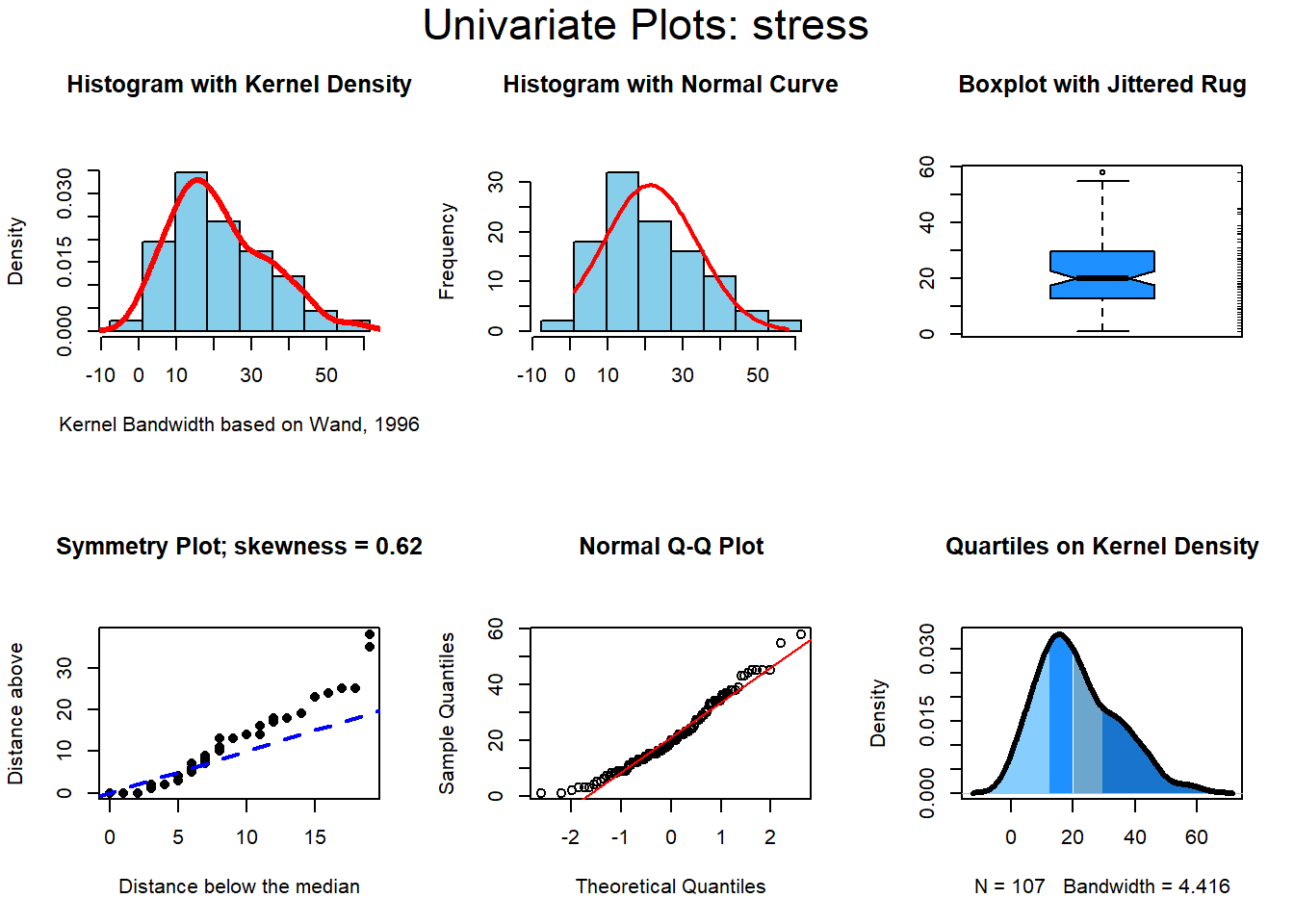## vars n mean sd median trimmed mad min max range skew kurtosis se ## X1 1 107 21.29 12.49 20 20.49 11.86 1 58 57 0.64 -0.08 1.21 The stress variable doesn’t look very much like it came from a normal distribution. It has a bit of positive skewness. The normal QQ plot from the explore function can also show this departure from normality, as does the kernel density function. But let’s introduce another graphing function here to reinforce this perspective. The qqPlot function in the car package gives us a nice normal probility plot that has “confidence bands”. This permits evaluation of the magnitude of devation from normality by asking how many data points fall outside the 95% bands. For the stress variable, this is actually smaller than the 5% of the 107 cases we might expect. So, the departure from normality is not severe. qqPlot(stress, id=F, distribution="norm")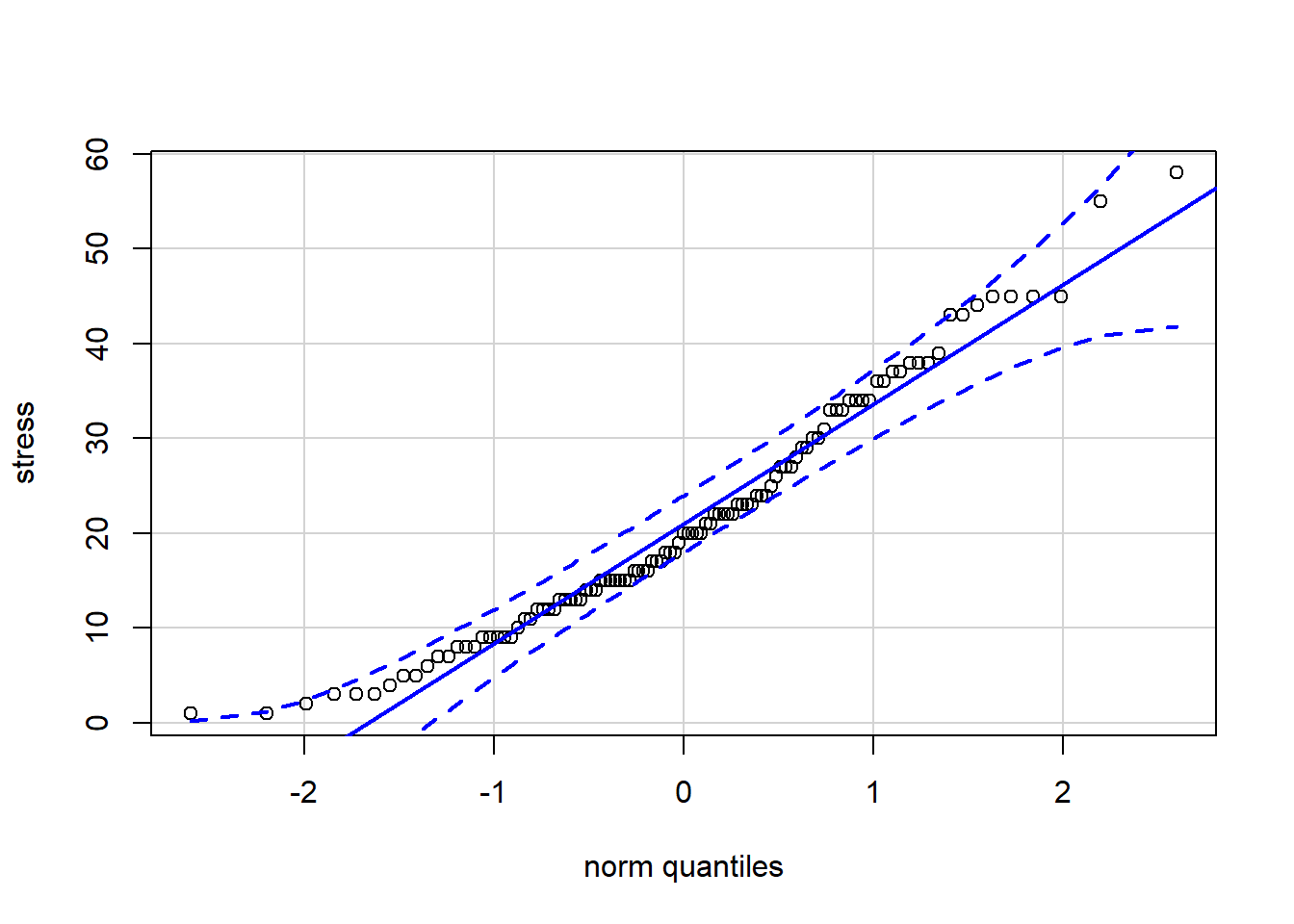## 4.2 EDA for the symptoms variable explore(symptoms)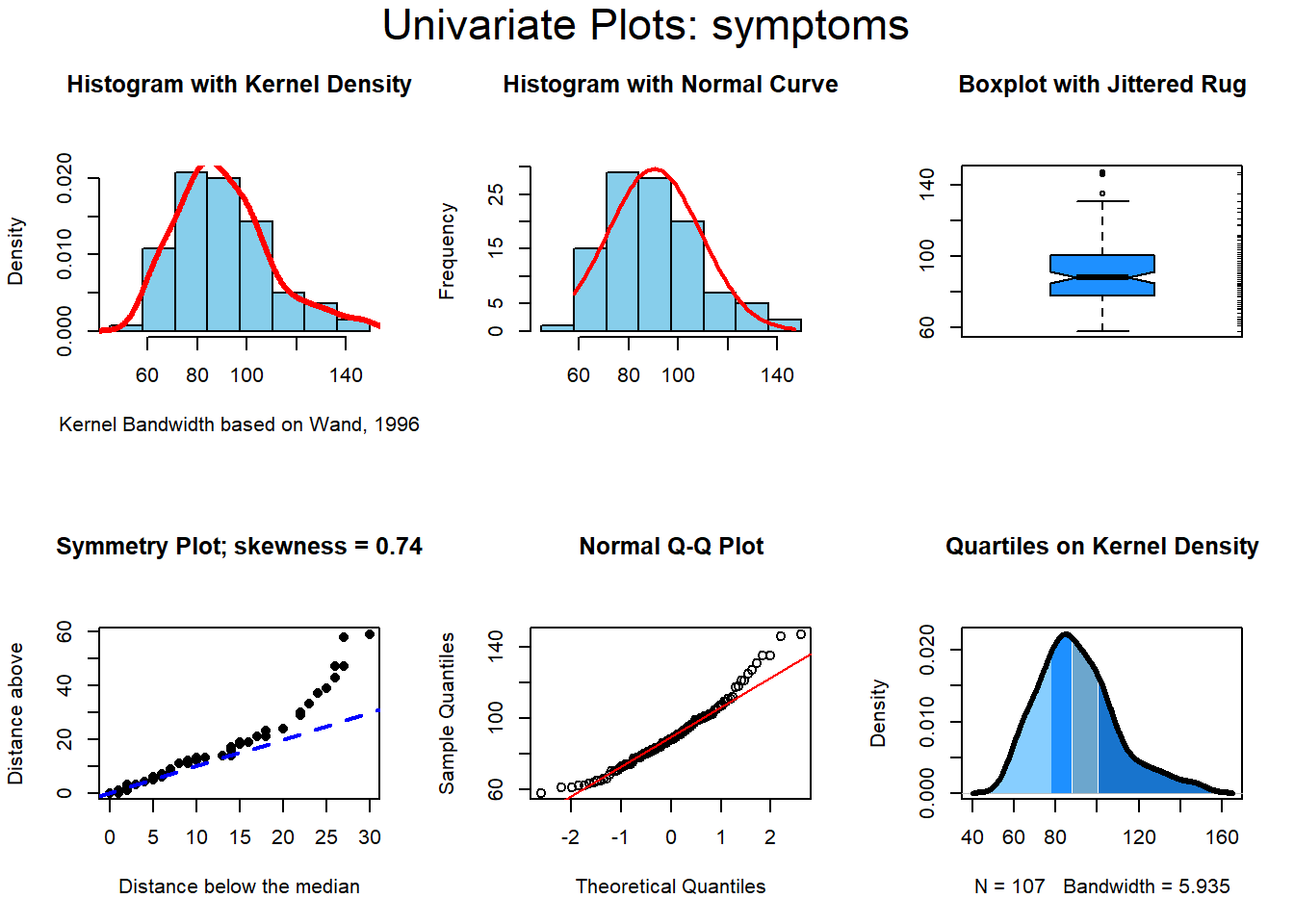## vars n mean sd median trimmed mad min max range skew kurtosis se ## X1 1 107 90.33 18.81 88 88.87 17.79 58 147 89 0.77 0.6 1.82 And the qqPlot application to the symptoms variable…… qqPlot(symptoms, id=FALSE, distribution="norm")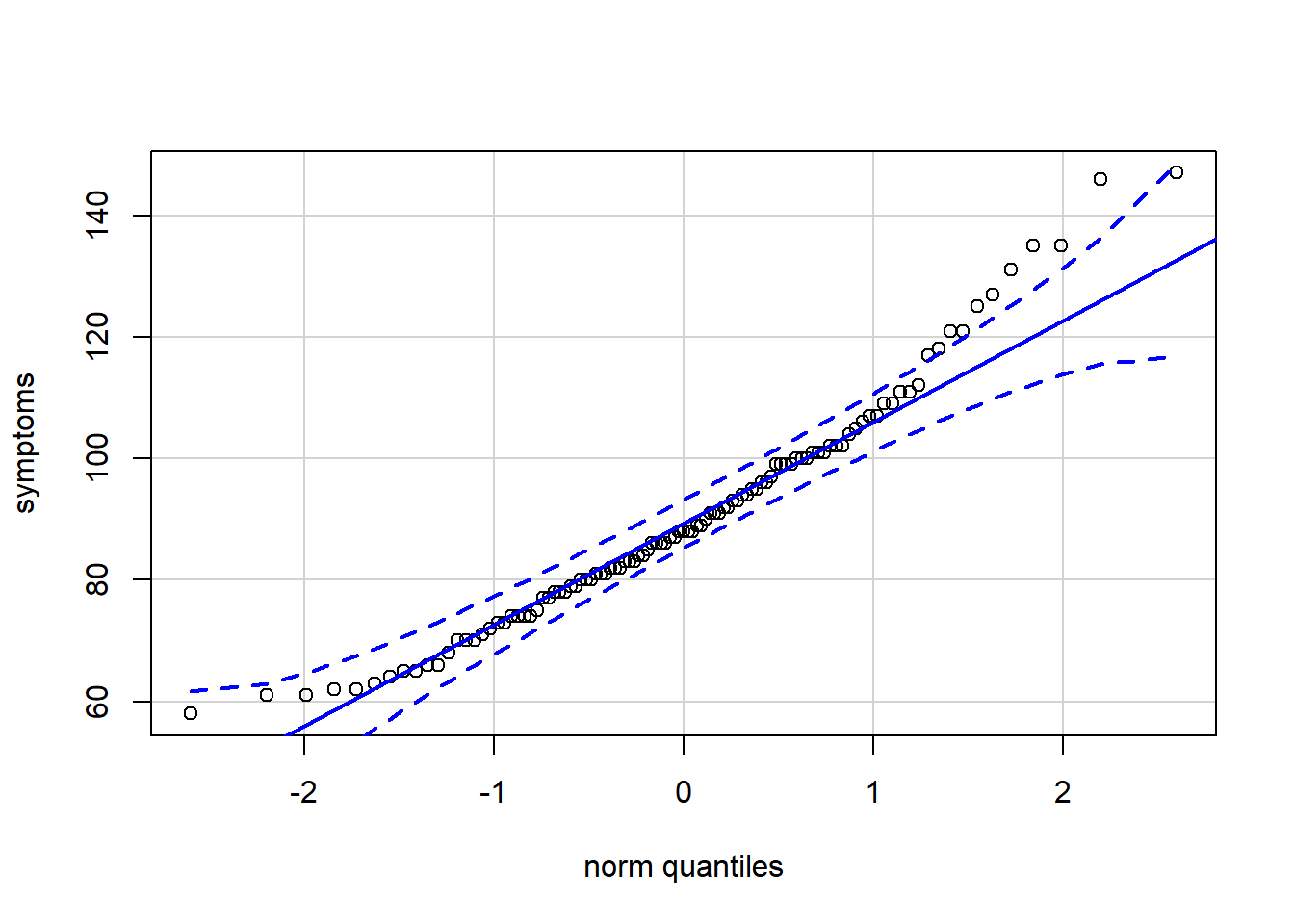Symptoms may be a bit more positively skewed, but neither variable are horrible departures from normality. Nonetheless, we may want to be careful to pay attention to the assumptions for tests of correlation and regression below. # 5 Bivariate Scatterplots There are many ways in R to generate bivariate scaterplots. Another document on “Scatterplots in R” has been created to show many of these possibilities. It can be found elsewhere in other class materials. Here, we will use one interesting approach with base system graphics that gives the scatterplot, rugplots for each variable, and boxplots in the respective margins for the two variables. A scatterplot can be drawn with the most basic of R graphing functions, the plot function. Everything else shown here is an add-on and not really necessary. Nonetheless, this additinal complexity of the figure creates a nice product that shows a great deal of information. PLEASE REALIZE that you can draw a similar graph of any two variables (your own data) simply by changing the variable names to those of your data set (and the axis and graph title labels). #win.graph(8,6) par(fig=c(0,0.8,0,0.8)) plot(stress, symptoms, xlab="Perceived Stress", ylab="Mental Health Symptoms") abline(lm(symptoms~stress, data=data1), col="red") # regression line (y~x) rug(symptoms,side=4,col="grey") rug(jitter(stress),side=1,col="grey") par(fig=c(0,0.8,0.55,1), new=TRUE) boxplot(stress, horizontal=TRUE, axes=FALSE) par(fig=c(0.65,1,0,0.8),new=TRUE) boxplot(symptoms,axes=FALSE) mtext("Scatterplot & Linear Regression Plus Univariate Boxplots", side=3, outer=TRUE, line=-3)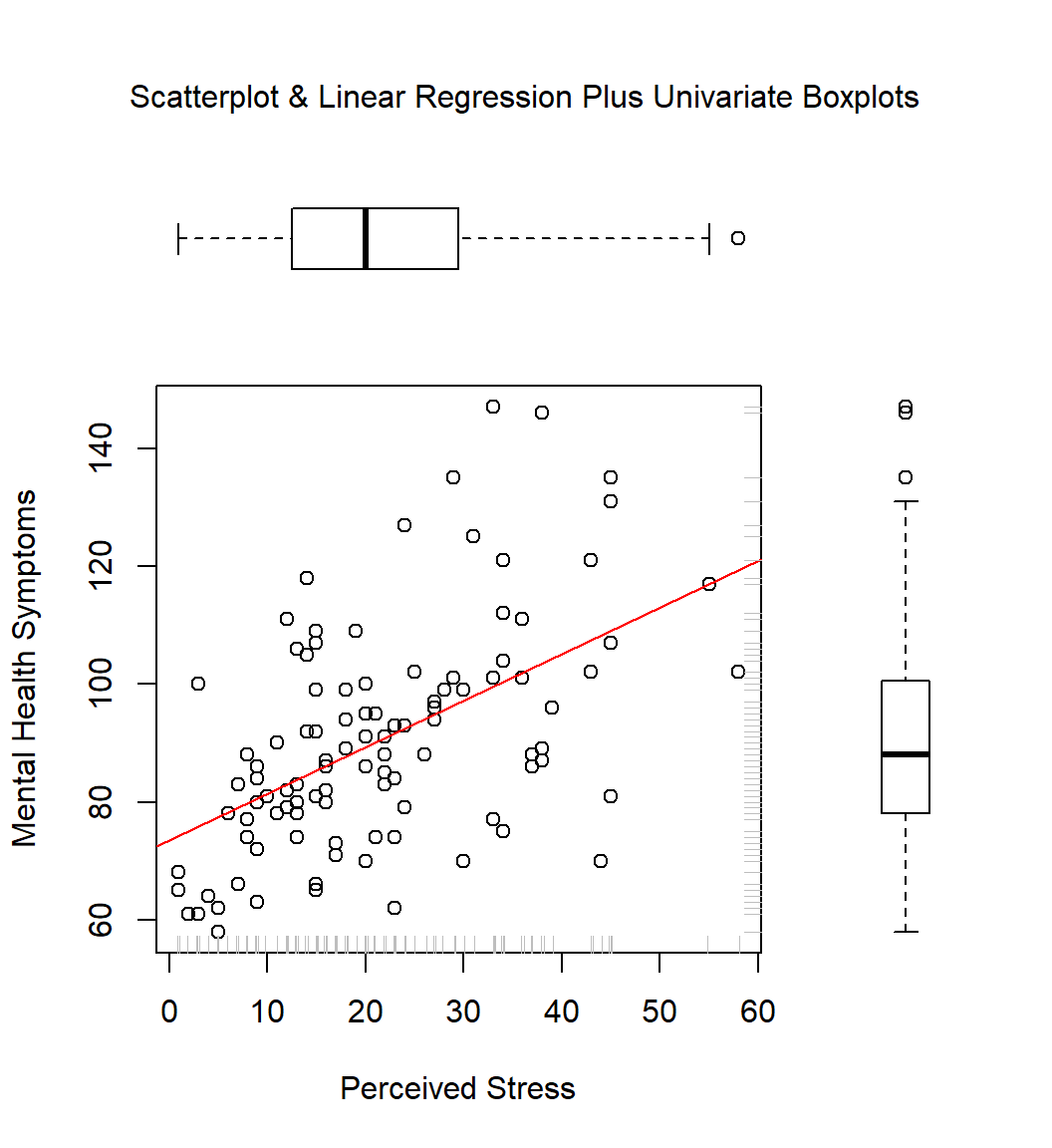# 6 Bivariate Corelation. Covariance and the Pearson product-moment correlation coefficient. R provides basic functions for covariance and correlation as well as a test of the pearson product-moment correlation using the t-test that we learned. First, the covariance……. We can pass the two variables of interest, or we could submit a data frame with larger numbers of variables and a variance-covariance matrix will be returned. cov(symptoms,stress) ##  123.2817 And the Pearson product-moment correlation coefficient….. cor(symptoms, stress) ##  0.5247429 The cor.test function gives a test of a two-tailed alternative hypothesis. One sided tests can be specified by changing the “alternative” argument. In addition, tests of non-parametric correlation coefficients (Kendal’s tau and Spearman’s Rho) can also be specified. See the help page (?cor.test) or a later section of this document. cor.test(symptoms,stress) ## ## Pearson's product-moment correlation ## ## data: symptoms and stress ## t = 6.3165, df = 105, p-value = 6.559e-09 ## alternative hypothesis: true correlation is not equal to 0 ## 95 percent confidence interval: ## 0.3719397 0.6498580 ## sample estimates: ## cor ## 0.5247429 To illustrate using cov and cor to produce Variance-Covariance and Correlation matrices, we use the built-in R data set called mtcars. It is a data set on performance specs of several makes of automobiles. data(mtcars) kable(head(mtcars)) mpg cyl disp hp drat wt qsec vs am gear carb Mazda RX4 21.0 6 160 110 3.90 2.620 16.46 0 1 4 4 Mazda RX4 Wag 21.0 6 160 110 3.90 2.875 17.02 0 1 4 4 Datsun 710 22.8 4 108 93 3.85 2.320 18.61 1 1 4 1 Hornet 4 Drive 21.4 6 258 110 3.08 3.215 19.44 1 0 3 1 Hornet Sportabout 18.7 8 360 175 3.15 3.440 17.02 0 0 3 2 Valiant 18.1 6 225 105 2.76 3.460 20.22 1 0 3 1 To keep the illustration simple, let’s extract a few of the quantitative variables from this data set - some are more reasonably looked at as as factors and we will leave them out. The filter function permits specification of a subset of columns from mtcars. The commented out line of code shows how to do the same thing with base R code (See the accompanying document on subsetting in R for more detail). mtcars2 <- dplyr::select(mtcars, mpg, disp, hp, drat, wt) #mtcars2 <- mtcars[,c(1,3:6)] kable(headTail(mtcars2)) mpg disp hp drat wt Mazda RX4 21 160 110 3.9 2.62 Mazda RX4 Wag 21 160 110 3.9 2.88 Datsun 710 22.8 108 93 3.85 2.32 Hornet 4 Drive 21.4 258 110 3.08 3.21 Ford Pantera L 15.8 351 264 4.22 3.17 Ferrari Dino 19.7 145 175 3.62 2.77 Maserati Bora 15 301 335 3.54 3.57 Volvo 142E 21.4 121 109 4.11 2.78 Now we can see how cov and cor handle larger numbers of variables. The result from the cov function is a variance-covariance matrix with variances of the variables on the leading diagonal and covariances off-diagonal. Note that it is a symmetrical matrix. # use the round function to control the number of decimal places displayed. cov2 <- round(cov(mtcars2),digits=3) gt(cov2) # check that the diagonal contains variances #var(mtcars2$wt)
mpg disp hp drat wt
36.324 -633.097 -320.732 2.195 -5.117
-633.097 15360.800 6721.159 -47.064 107.684
-320.732 6721.159 4700.867 -16.451 44.193
2.195 -47.064 -16.451 0.286 -0.373
-5.117 107.684 44.193 -0.373 0.957

A correlation matrix is a standardized covariance matrix, and is also symmetrical.

# use the round function to control the number of decimal places displayed.
cor2 <- round(cor(mtcars2),digits=2)
gt(cor2)
mpg disp hp drat wt
1.00 -0.85 -0.78 0.68 -0.87
-0.85 1.00 0.79 -0.71 0.89
-0.78 0.79 1.00 -0.45 0.66
0.68 -0.71 -0.45 1.00 -0.71
-0.87 0.89 0.66 -0.71 1.00

# 7 Simple Regression

Prediction of symptoms from stress levels requires an R function that is called lm. Much like the SPSS regression procedure, it is capable of expanding to larger number of IVs in multiple regression and other uses. Here, we simply specify symptoms as the DV and stress as the IV, using a model syntax that you have seen before, using the tilda symbol (~).

Unlike SPSS, the lm function itself only generates the model object. Other functions are required to obtain the detailed summary information. The two additional basic functions are anova and summary. More detailed work will follow below the basics presented here in this section.

The summary function produces a table with the estimates of the regression coefficients, and their standard errors, along with the t-tests for both the intercept and the slope. The test of the intercept is a test of the null that the intercept is zero. The Residual Standard error is the square root of MSresidual (see the Anova summary table below).

# fit the model and save it as an objec that we choose to call fit1
fit1 <- lm(symptoms~stress)
# summarize the model
summary(fit1)
##
## Call:
## lm(formula = symptoms ~ stress)
##
## Residuals:
##     Min      1Q  Median      3Q     Max
## -38.270 -11.222  -0.778   7.037  47.421
##
## Coefficients:
##             Estimate Std. Error t value Pr(>|t|)
## (Intercept)  73.5066     3.0838  23.837  < 2e-16 ***
## stress        0.7901     0.1251   6.317 6.56e-09 ***
## ---
## Signif. codes:  0 '***' 0.001 '**' 0.01 '*' 0.05 '.' 0.1 ' ' 1
##
## Residual standard error: 16.09 on 105 degrees of freedom
## Multiple R-squared:  0.2754, Adjusted R-squared:  0.2685
## F-statistic:  39.9 on 1 and 105 DF,  p-value: 6.559e-09

We will need to request the confidence interval for the regression coefficient. The confint function provides one for the interecept also.

#obtain a 95%CI for the regression coefficients
confint(fit1, level=0.95)
##                  2.5 %    97.5 %
## (Intercept) 67.3920818 79.621162
## stress       0.5420636  1.038087

The anova function gives the SS partitioning and the same basic ANOVA summary table we saw in SPSS. Note that the F value is the same as above, and the square of the t for the test of the regression coefficient.

anova(fit1)
## Analysis of Variance Table
##
## Response: symptoms
##            Df Sum Sq Mean Sq F value    Pr(>F)
## stress      1  10325 10324.6  39.899 6.559e-09 ***
## Residuals 105  27171   258.8
## ---
## Signif. codes:  0 '***' 0.001 '**' 0.01 '*' 0.05 '.' 0.1 ' ' 1

## 7.1 Diagnostic plots for Residual assumptions

R provides a direct and simple method to obtain several important diagnostic plots for regression objects. The two figures on the left are similar to those we obtained from SPSS. The two on the right involve things we will get to next semester. The plot function is aware of model objects that are lm fits. It “knows” to generate the following four types of graphs simply by passing the lm fit object to plot. The first plot suggests the presence of some heteroscedasticity. With larger yhat values, the spread in the residuals is slightly larger. We will return to this in a later section, and look at tests of homoscedasticity. The normal QQ plot does suggest some non-normality of the residuals and we will also examine this further in the later sections.

layout(matrix(c(1,2,3,4),2,2)) # optional 4 graphs/page
plot(fit1)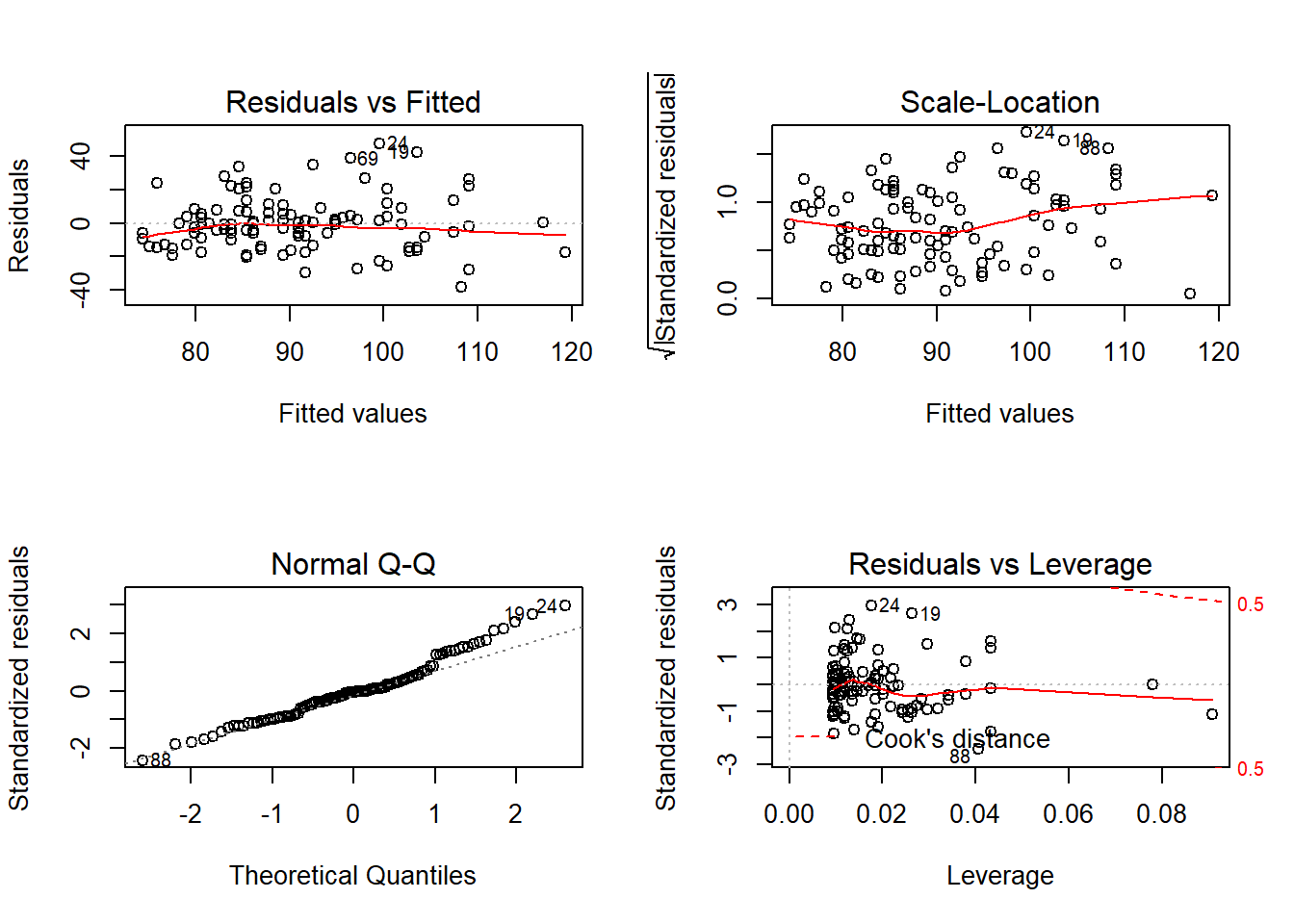## 7.2 We can extract the residuals and yhats from the model object and work with them directly

In SPSS we viewed the scatterplot of residuals against predicted in standardized form rather than raw scale form as was the case just above. We might also want to obtain a frequency histogram of the residuals, and perhaps more sophisiticated plots to evaluate the residuals. This section shows how to extract residuals and yhats from a regression model fit object, a method to standardize them, and use of them in plots to evaluate the normality and homoscedasticity assumptions.

## work with residuals and predicted scores a bit more
# create new vectors for residuals/yhats, also standardized
# and then add them to the original data frame by
# using the cbind function
fit1.resid <- residuals(fit1)
fit1.pred <- predict(fit1)
# we can use the scale funciton in R to
# center and standardize variables.  Here, I standardize.
fit1.zresid <- scale(fit1.resid, scale=T)
fit1.zpred <- scale(fit1.pred, scale=T)
detach(data1)  # need to detach it before adding in the new vars
# use the "column bind" function to add the newly created variables to the original data frame.
data2 <- cbind(data1,fit1.resid,fit1.pred,fit1.zresid,fit1.zpred)

Next, examine the new data frame which now contains the raw and standardized residuals and yhats (predicted scores).

# look at the first few lines of the new data frame
# attach it for use below
attach(data2)
## The following objects are masked _by_ .GlobalEnv:
##
##     fit1.pred, fit1.resid, fit1.zpred, fit1.zresid
##   id stress symptoms  fit1.resid fit1.pred fit1.zresid fit1.zpred
## 1  1     30       99   1.7911200  97.20888  0.11187296  0.6972958
## 2  2     27       94  -0.8386541  94.83865 -0.05238215  0.4571328
## 3  3      9       80  -0.6172992  80.61730 -0.03855637 -0.9838455
## 4  4     20       70 -19.3081272  89.30813 -1.20598139 -0.1032477
## 5  5      3      100  24.1231525  75.87685  1.50672681 -1.4641716
## 6  6     15      109  23.6422492  85.35775  1.47668969 -0.5035194

Now we can use those newly extracted/created variables to draw useful plots and perform other analyses. We have seen each of these types of plots previously. Taken together they suggest a possible normality issue with the residuals. Some heteroscedasticity might be present since there is a bit higher spread of the residuals at higher yhat values

#win.graph(8,6)
layout(matrix(c(1,3,2,4,5,6),2,3)) #optional multiple graphs/figure
boxplot(fit1.resid,xlab="Residuals",data=data2,col="lightgrey")
boxplot(fit1.pred, xlab="Yhat",data=data2,col="lightgrey")
hist(fit1.zresid,breaks=12,prob=T)
lines(density(fit1.zresid),col = "red",lwd = 3)
hist(fit1.zpred,breaks=12,prob=T)
lines(density(fit1.zpred),col = "red",lwd = 3)
plot(fit1.zpred,fit1.zresid)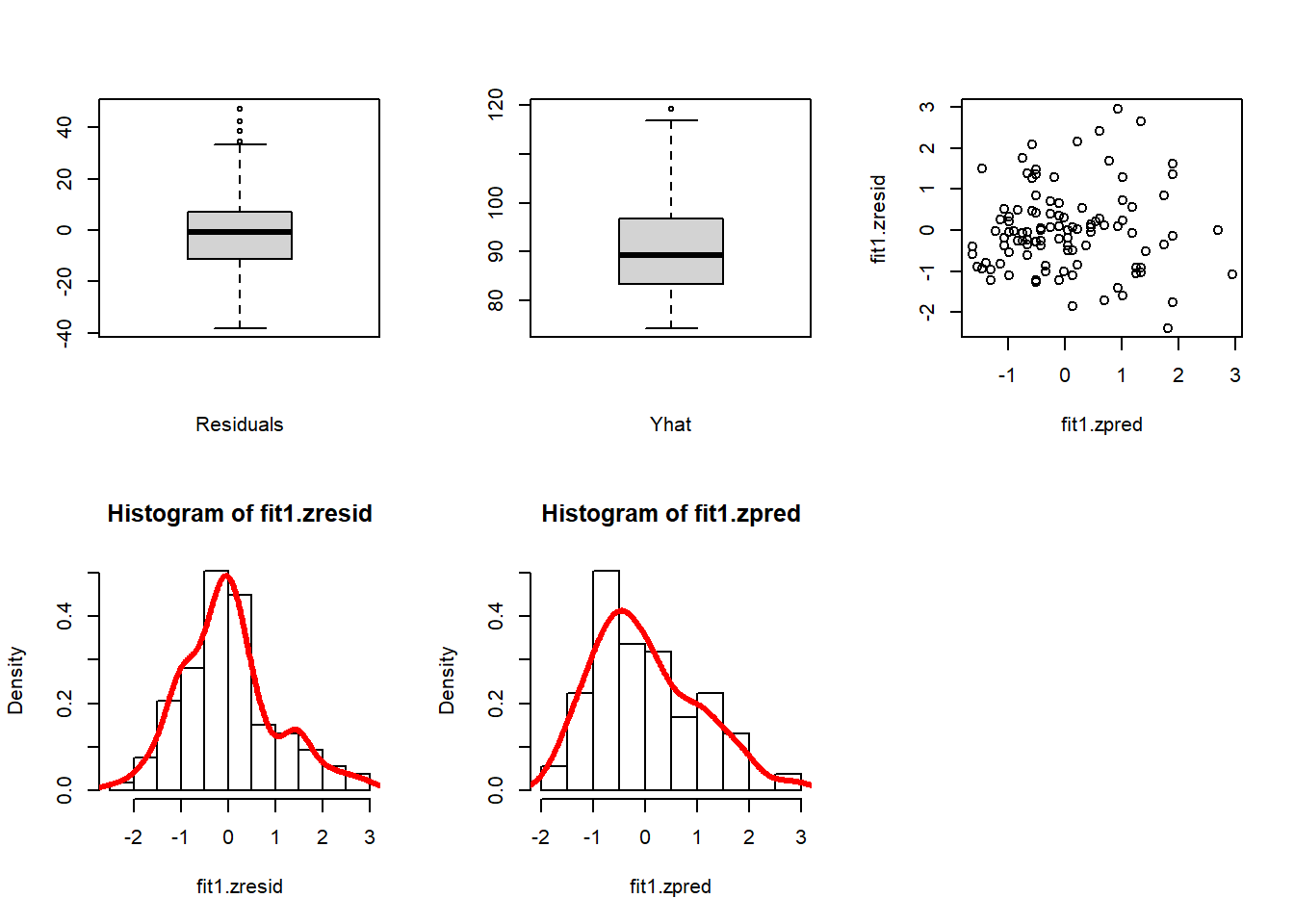The histogram of the residuals shows some slight positive skew (.54, seen from the numerical calculation below). A more interesting plot to examine the fit of the residuals to a normal distribution is to overlay a normal distribution curve onto the frequency histogram - This plot examines the standardized residuals, but the raw residuals would serve just as well. This simple function from the rcompanion package is useful in many different circumstances as well.

#library(rcompanion)
plotNormalHistogram(fit1.zresid)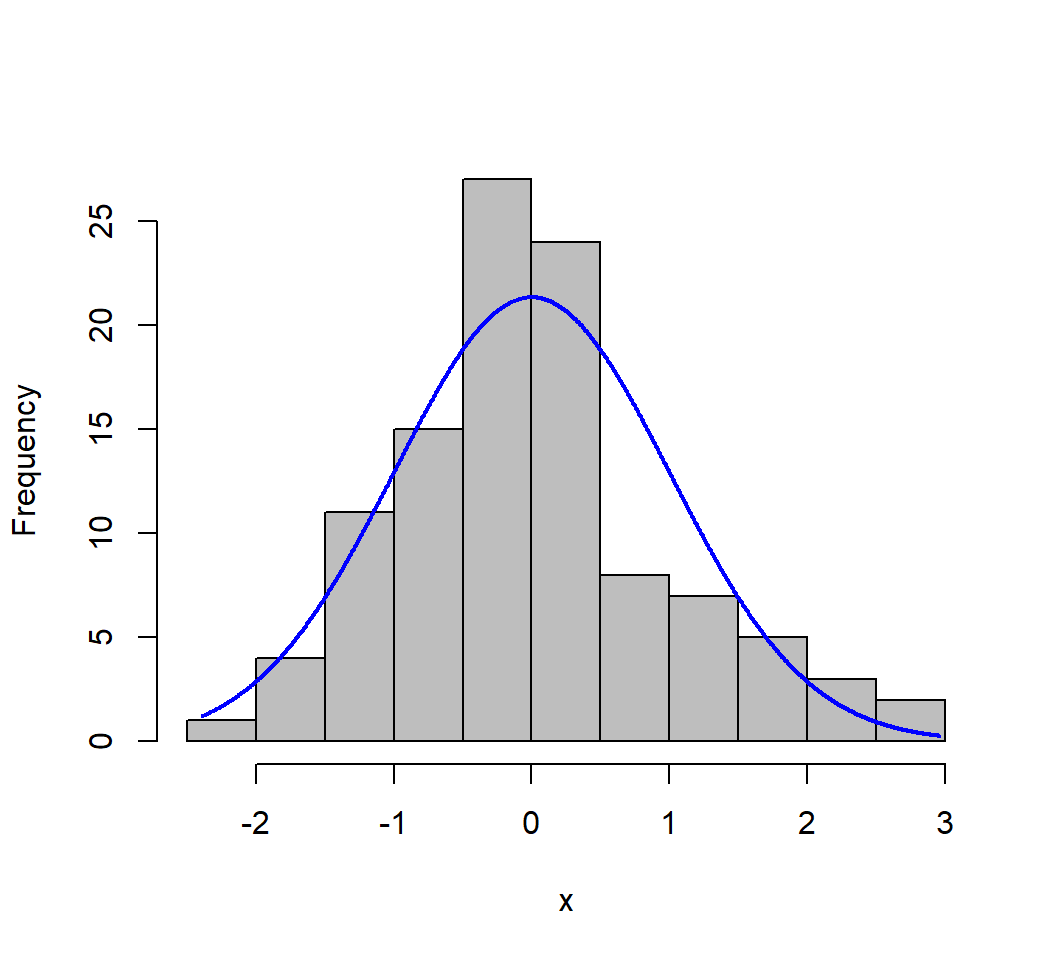It is also possible to obtain numerical summary info on these new varaibles (and all original ones) using describe from the psych package. The residuals are slightly positively skewed. We might consider a scale transformation to remedy this

kable(round(describe(data2,type=2), digits=2))
vars n mean sd median trimmed mad min max range skew kurtosis se
id 1 107 54.00 31.03 54.00 54.00 40.03 1.00 107.00 106.00 0.00 -1.20 3.00
stress 2 107 21.29 12.49 20.00 20.49 11.86 1.00 58.00 57.00 0.64 -0.08 1.21
symptoms 3 107 90.33 18.81 88.00 88.87 17.79 58.00 147.00 89.00 0.77 0.60 1.82
fit1.resid 4 107 0.00 16.01 -0.78 -0.87 12.72 -38.27 47.42 85.69 0.56 0.49 1.55
fit1.pred 5 107 90.33 9.87 89.31 89.70 9.37 74.30 119.33 45.03 0.64 -0.08 0.95
fit1.zresid 6 107 0.00 1.00 -0.05 -0.05 0.79 -2.39 2.96 5.35 0.56 0.49 0.10
fit1.zpred 7 107 0.00 1.00 -0.10 -0.06 0.95 -1.62 2.94 4.56 0.64 -0.08 0.10

## 7.3 Visually Examine the yhats

Now that we have extracted the yhats (predicted scores), we can revisit the initial bivariate scatterplot and redraw it with additional information. Displaying the yhats as a rug plot gives a useful visual perspective.

par(fig=c(0,0.8,0,0.8))
rangex <- max(data2$stress)- min(data2$stress)
rangey <- max(data2$symptoms)- min(data2$symptoms)
lft <- min(data2$stress)-(.08*rangex) rght <- max(data2$stress)+(.03*rangex)
yhatpos <- max(data2$stress)+(.055*rangex) plot(stress, symptoms, xlab="Stress", ylab="GSR", xlim=c(lft,rght)) abline(lm(symptoms~stress), col="black") # regression line (y~x) rug(jitter(symptoms),side=4,col="black") rug(jitter(stress),side=1) rug(jitter(fit1.pred),side=4,col="red", pos=yhatpos) text((min(data2$stress) + (.75*rangex)),
(min(data2\$symptoms)+(.05*rangey)),
paste("Red Rugplot is Yhat values"), cex=.7, col="red")
par(fig=c(0,0.8,0.55,1), new=TRUE)
boxplot(stress, horizontal=TRUE, axes=FALSE)
par(fig=c(0.65,1,0,0.8),new=TRUE)
boxplot(symptoms,axes=FALSE)
mtext("Scatterplot & Linear Regression Plus Univariate Boxplots", side=3, outer=TRUE, line=-3)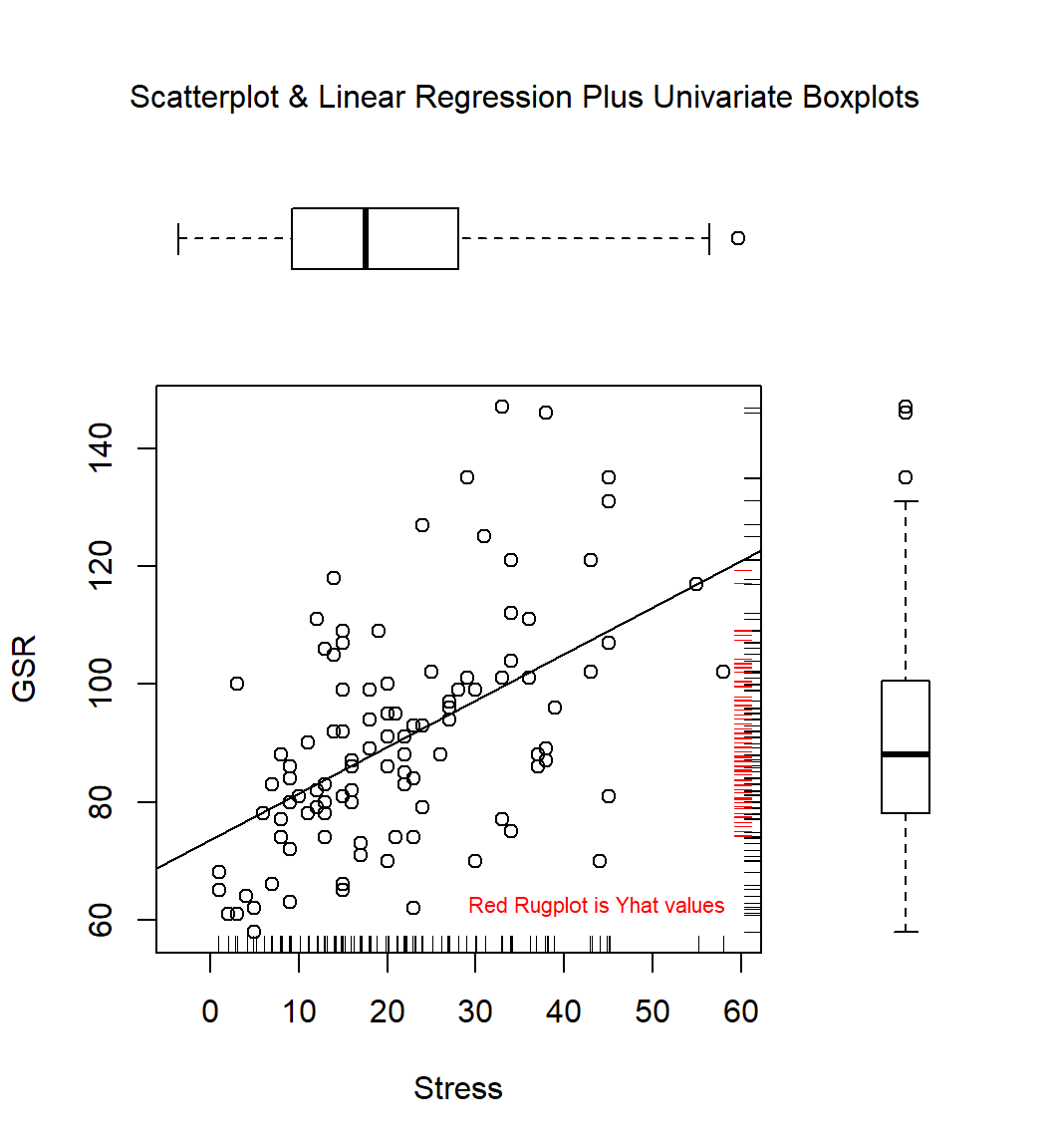Notice that the yhats are not as dispersed as the Y values. This is to be expected and reflects the “regression to the mean” typical of OLS. When the correlation is 1.0, then the yhats will match the Y values. When the xy correlation is zero, then there is now variation in the yhats; they will all equal the mean of Y. Also recall that $${{r}_{y\overset{\scriptscriptstyle\frown}{y}}}={{r}_{xy}}$$. The reader can explore yhat distributions, interactively, in this shiny app (usable in the html version of this document, but not visible in the pdf version:

## 7.4 Further evaluation and tests of Residual Homoscedasticity and Normality

The plot of residuals against yhats was generated above with the plot(fit1) code. With the extracted zresids and zpreds, we can generate that plot quickly. The plot gives somewhat of a hint that the residual variance might be heteroscedastic. For positive yhat values, the residual variation (vertical spread in the graph) seems to be larger. However, below, we will examine a way to test it.

plot(fit1.zpred, fit1.zresid)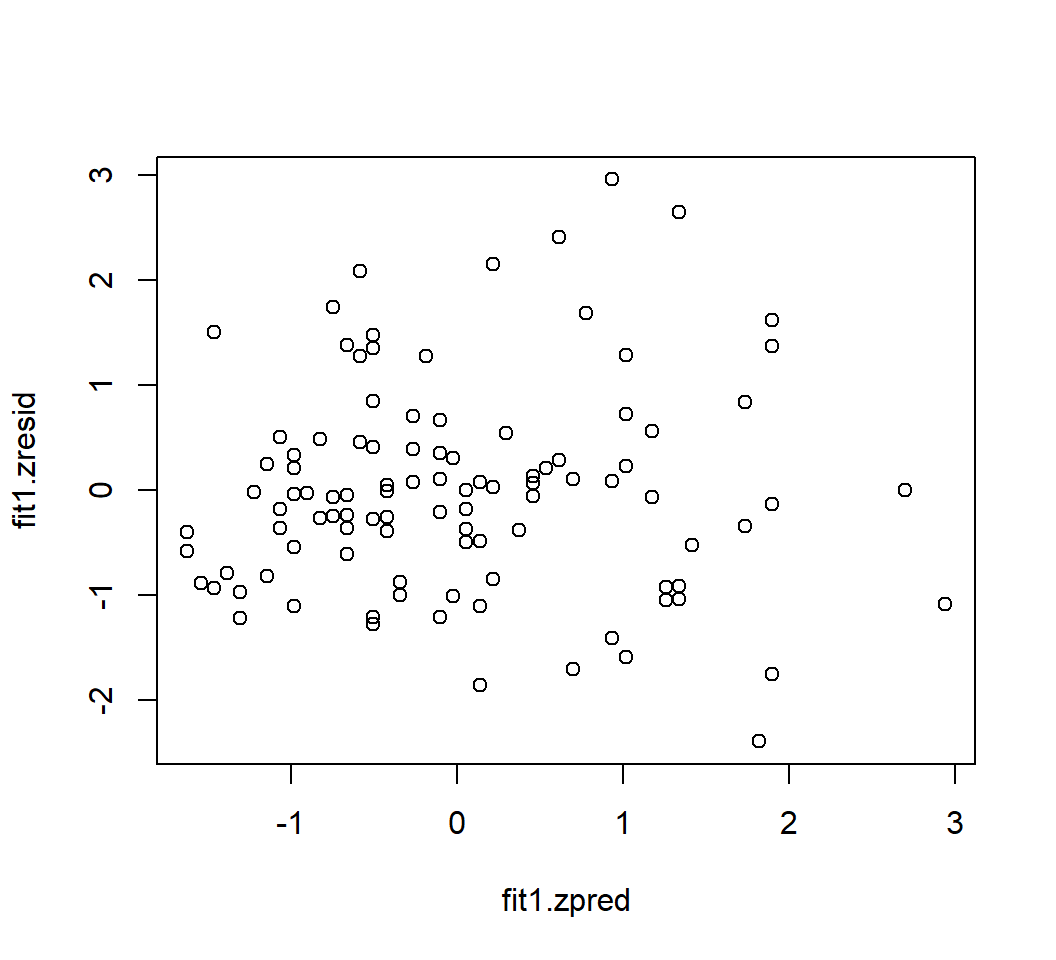Testing the homoscedasticity assumption in R can be accomplished several ways. The two most common are the Brausch-Pagan test, and the NCV test (non-constant variance)

lmtest::bptest(fit1)
##
##  studentized Breusch-Pagan test
##
## data:  fit1
## BP = 6.1457, df = 1, p-value = 0.01317
car::ncvTest(fit1)
## Non-constant Variance Score Test
## Variance formula: ~ fitted.values
## Chisquare = 7.407101, Df = 1, p = 0.0064967

Testing the assumption of residual normality can be accomplished with many different tests in R. One very commonly used test is the Shapiro-Wilk test. The slight non-normality visualized with the frequency histogram of the residuals and skewness computation above is found to be significant with this test. We can also test the original DV.

shapiro.test(fit1.resid)
shapiro.test(symptoms)
##
##  Shapiro-Wilk normality test
##
## data:  fit1.resid
## W = 0.97043, p-value = 0.01727
##
##
##  Shapiro-Wilk normality test
##
## data:  symptoms
## W = 0.95918, p-value = 0.00232

A test of normality that has been highly recommended is the anderson-darling test and it is available in the **** package.

#library(nortest)
ad.test(fit1.resid)
##
##  Anderson-Darling normality test
##
## data:  fit1.resid
## A = 1.1929, p-value = 0.003932
ad.test(symptoms)
##
##  Anderson-Darling normality test
##
## data:  symptoms
## A = 0.93263, p-value = 0.01738

# 8 Conclusions about assumptions and remedies

Even though the degree of heteroscedasticity and residual non-normality was not extreme, there is some indication that these crucial assumptions might not be satisfied in the bivariate population system of these two variables. It is important to be able to evaluate these assumptions both graphically and inferentially. But what options does one have if the assumptions are violated? Several possibilities exist: scale transformation of the DV and perhaps the IV; Robust methods; resampling methods such as bootstrapping. Sections on these topics are found below.

## 8.1 Influence Analysis

Foreshadowing things we will do next semester, we can obtain statistics on “influence analysis” for this model. This simply introduces the notion that influence analysis in Multiple regression is an integral part of the basic analysis and is included here as a placeholder to remind us of that next semester. The influence.measures function provides several indices of influence - the output is long so is not included here. The influencePlot function from car provides a revision of the residuals vs yhat plot that scales the points to the size of influence for that case.

# This is a standard plot of residuals agains yhats.
# The area of the points is proportional to the degree of influence of each case and
# this topic will be covered later.  Cook's D is used to scale the points.
#influence.measures(fit1)
influencePlot(fit1)

# 9 Scale transformations

Two general options for scale transformations exist. One is to search for scale transformations of Y and X that best produce a sample distribution that approximates a normal. This might implement usage of Tukey’s “ladder of powers” or it might be based on more advanced criteria. A second major approach is to use the Box-Cox transformation algorithm.

Historically, use of transformations in regression is most commonly applied to situations where positive skewness exists in the variables, at times a result of the properties of the measurement instrument. Researchers have most often resorted to using either a square root transformation or a base 10 log transformation. At times other transformations are tried, including natural logs and other fractional exponents, implementing Tukey’s ladder of powers, including fractional and negative powers (e.g., an exponent of .5 gives the square root, but a -.5 could also be considered). These have typically been chosen with a trial and error approach. Some new capabilities in R have attempted to automate this approach and one is outlined here.

The modern data science world has a need for normalizing variables that are used in machine learning predictive techniques (which are ultimately an extensive elaboration of simple regression to multiple predictors in large data sets with many potential IVs). There is an R package that implements several approaches to finding good scale transformations for dependent and independent variables (sometimes called covariates in the data science world). One package attempts to put together several different approaches and compare them to produce a recommendation about the best transformation. The goal is to produce a scale change that yeilds the best fit to a normal distribution. Use of this bestNormalize functions should only be done after a careful reading of the package vignette.

https://cran.r-project.org/web/packages/bestNormalize/vignettes/bestNormalize.html

These approaches are atheoretical and as such are subject to criticism. Some caution is also advised since results of this illustration can change if the random number seed is changed. Smiply re-running this same code without setting the seed can produce a different recommendation. Nonetheless, it is an interesting possibility to save time and consider many possible transformations.

The bestNormalize function works on one variable at a time and the DV is illustrated here. I have set the random number seed here to produce a replicable outcome for purposes of this document. Note that the best recommendation for transforming the scale of the DV (symptoms) is actually a square root transformation. Comparative information for a larger class of posssible transformations is also provided.

#install.packages("bestNormalize")
#library(bestNormalize)
set.seed(12354)
bestNormalize(symptoms, allow_lambert_s = T)
## Best Normalizing transformation with 107 Observations
##  Estimated Normality Statistics (Pearson P / df, lower => more normal):
##  - No transform: 1.0762
##  - Box-Cox: 1.1417
##  - Lambert's W (type s): 1.1635
##  - Log_b(x+a): 1.0232
##  - sqrt(x+a): 0.9366
##  - exp(x): 12.379
##  - arcsinh(x): 1.0232
##  - Yeo-Johnson: 1.1417
##  - orderNorm: 1.2064
## Estimation method: Out-of-sample via CV with 10 folds and 5 repeats
##
## Based off these, bestNormalize chose:
## Standardized sqrt(x + a) Transformation with 107 nonmissing obs.:
##  Relevant statistics:
##  - a = 0
##  - mean (before standardization) = 9.455023
##  - sd (before standardization) = 0.9687161
#bestNormalize::boxcox(symptoms)
#bestNormalize(stress)

The Box-Cox tranformation capability is typically not applied to a single variable in isolation. Most commonly, the Box-Cox approach is designed to find a transformation of the DV that best results in residual normality of a regression model. So, an lm fit object is passed to the boxCox function - several packages in R permit this approach and the one used here is from the car package. In regression, the critical normality assumption is normality of the residuals. Although normality of both IV and DV in a bivariate normality distribution is sufficient to produce residual normality, it is not necessary. It is the DV distribution shape that most directly influences the residual distribution shape. Thus the IV distribution normality is typically not an issue of interest in regression work.

The function produces a graph that is not interpretable without some explanation. The x axis reflects various possible $$\lambda$$ values to be used in the transformation. The actual transformation is

${{y}_{BCtransformed}}=\frac{{{y}^{\lambda }}-1}{\lambda }$

If $$\lambda$$ is zero, then a base 10 log transform is used.

This approach assumes that all Y values are positive. If some/all y’s are negative the user could simply add the minimum value (plus 1) to all scores. But most software implementations incorporate a Box/Cox recommendation for an alternative formulation when some Y values are negative.

The Y axis of the graph provides an index that reflects how well the $$\lambda$$ value produces a satisfactory regression fit with residual normality. In our example, the plot seems to peak when $$\lambda$$ is approximately -.25. But the plot also gives a range of values in which confidence is high that the $$\lambda$$ value produces reasonable normality. The points at which the parabola intersects this 95% confidence level provides a range of possible $$\lambda$$ values to use. It is usually recommended to use a “nice” value near some interpretable value such as a fraction. -.3 is close to -1/3 or -1/4, so those could be a good choice. But zero might be a better choice and that would result in doing a log 10 transformation. Some subjectivity is obviously involved.

# require(car)
boxCox(fit1)

But how can we find the actual exact recommended $$\lambda$$ from the peak of the curve. If we save the boxCox object, it is a data frame that has the x and y values. If we sort the data frame by the y axis values, we can extract the x value associated with that highest y value.

bc1 <- as.data.frame(boxCox(fit1))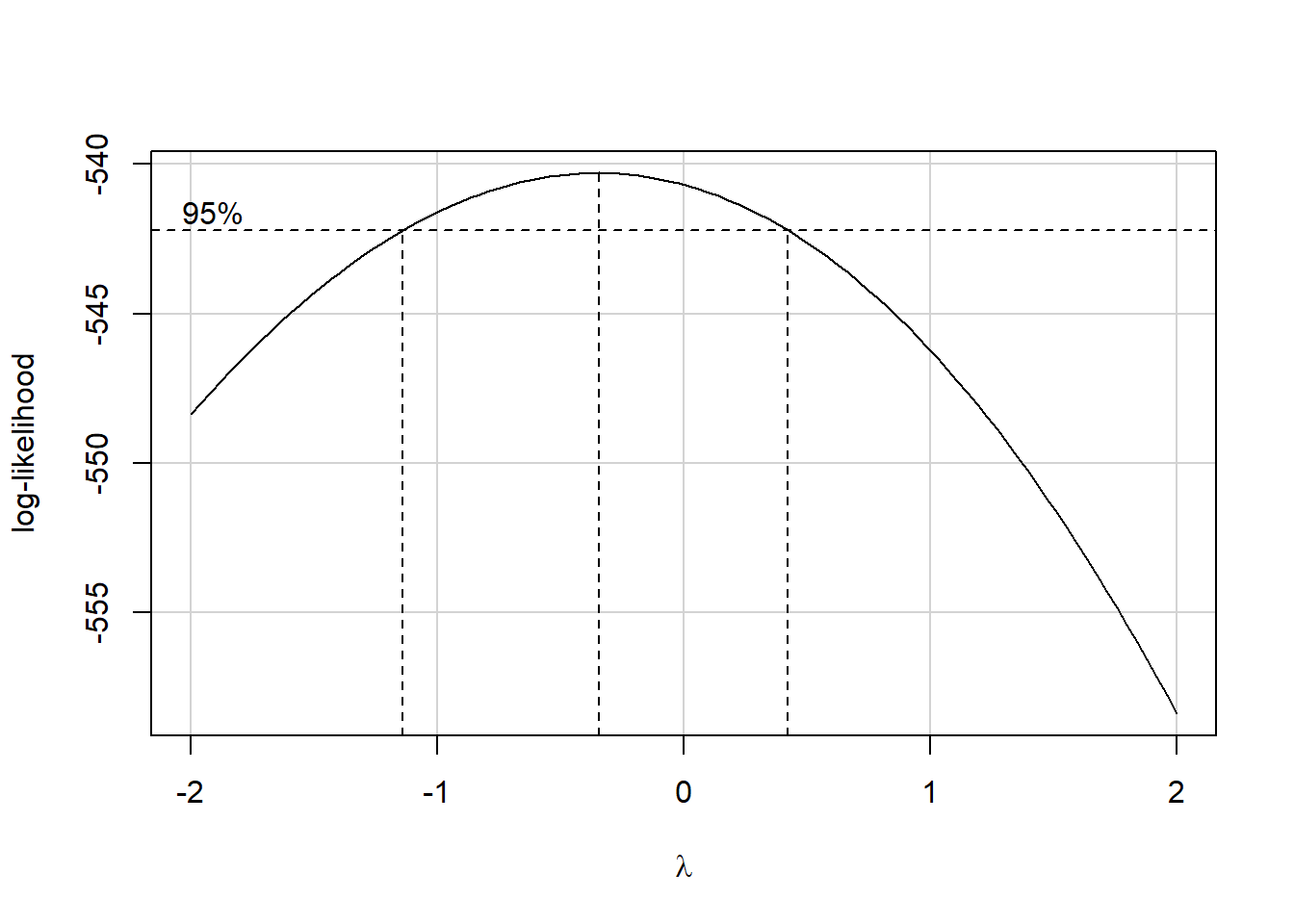bc1 <- dplyr::arrange(bc1, -y) # sort to smallest Y first
head(bc1)
##            x         y
## 1 -0.3434343 -540.2885
## 2 -0.3838384 -540.2921
## 3 -0.3030303 -540.2953
## 4 -0.4242424 -540.3059
## 5 -0.2626263 -540.3125
## 6 -0.4646465 -540.3300
lambda <-bc1[1,1]
lambda
##  -0.3434343

Next we can create a transformed symptoms variable that uses the $$\lambda$$ from the BoxCox analysis. If we create that variable and add it into the data frame, we can refit the regression model and examine the residuals.

tsymptoms <- ((symptoms^lambda)-1)/lambda
data3 <- cbind(data1,tsymptoms)
gt(head(data3))
id stress symptoms tsymptoms
1 30 99 2.310886
2 27 94 2.300096
3 9 80 2.265263
4 20 70 2.234924
5 3 100 2.312957
6 15 109 2.330420

Now refit the regression.

fit1bc <- lm(tsymptoms~stress, data=data3)
broom::tidy(fit1bc)
## # A tibble: 2 x 5
##   term        estimate std.error statistic   p.value
##   <chr>          <dbl>     <dbl>     <dbl>     <dbl>
## 1 (Intercept)  2.25     0.00707     318.   1.80e-158
## 2 stress       0.00183  0.000287      6.37 5.08e-  9

And now we can examine the residual distribution from the new regression analysis.

plotNormalHistogram(residuals(fit1bc))